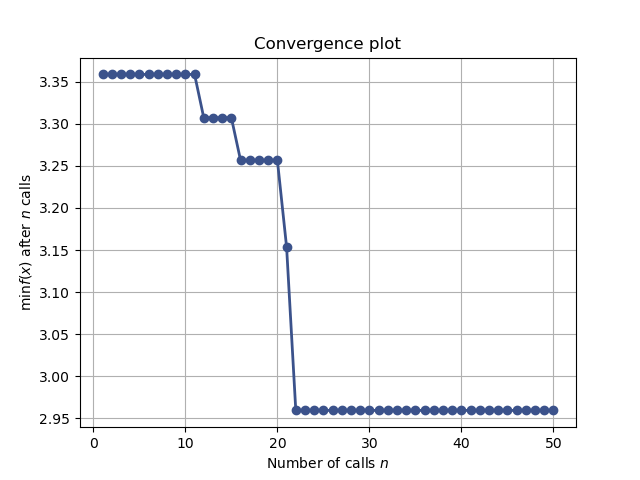# Tuning a scikit-learn estimator with skopt¶

Gilles Louppe, July 2016 Katie Malone, August 2016 Reformatted by Holger Nahrstaedt 2020

If you are looking for a sklearn.model_selection.GridSearchCV replacement checkout Scikit-learn hyperparameter search wrapper instead.

## Problem statement¶

Tuning the hyper-parameters of a machine learning model is often carried out using an exhaustive exploration of (a subset of) the space all hyper-parameter configurations (e.g., using sklearn.model_selection.GridSearchCV), which often results in a very time consuming operation.

In this notebook, we illustrate how to couple gp_minimize with sklearn’s estimators to tune hyper-parameters using sequential model-based optimisation, hopefully resulting in equivalent or better solutions, but within less evaluations.

Note: scikit-optimize provides a dedicated interface for estimator tuning via BayesSearchCV class which has a similar interface to those of sklearn.model_selection.GridSearchCV. This class uses functions of skopt to perform hyperparameter search efficiently. For example usage of this class, see Scikit-learn hyperparameter search wrapper example notebook.

print(__doc__)
import numpy as np


## Objective¶

To tune the hyper-parameters of our model we need to define a model, decide which parameters to optimize, and define the objective function we want to minimize.

from sklearn.datasets import load_boston
from sklearn.model_selection import cross_val_score

X, y = boston.data, boston.target
n_features = X.shape

# gradient boosted trees tend to do well on problems like this


Next, we need to define the bounds of the dimensions of the search space we want to explore and pick the objective. In this case the cross-validation mean absolute error of a gradient boosting regressor over the Boston dataset, as a function of its hyper-parameters.

from skopt.space import Real, Integer
from skopt.utils import use_named_args

# The list of hyper-parameters we want to optimize. For each one we define the
# bounds, the corresponding scikit-learn parameter name, as well as how to
# sample values from that dimension ('log-uniform' for the learning rate)
space  = [Integer(1, 5, name='max_depth'),
Real(10**-5, 10**0, "log-uniform", name='learning_rate'),
Integer(1, n_features, name='max_features'),
Integer(2, 100, name='min_samples_split'),
Integer(1, 100, name='min_samples_leaf')]

# this decorator allows your objective function to receive a the parameters as
# keyword arguments. This is particularly convenient when you want to set
# scikit-learn estimator parameters
@use_named_args(space)
def objective(**params):
reg.set_params(**params)

return -np.mean(cross_val_score(reg, X, y, cv=5, n_jobs=-1,
scoring="neg_mean_absolute_error"))


## Optimize all the things!¶

With these two pieces, we are now ready for sequential model-based optimisation. Here we use gaussian process-based optimisation.

from skopt import gp_minimize
res_gp = gp_minimize(objective, space, n_calls=50, random_state=0)

"Best score=%.4f" % res_gp.fun


Out:

'Best score=2.9593'

print("""Best parameters:
- max_depth=%d
- learning_rate=%.6f
- max_features=%d
- min_samples_split=%d
- min_samples_leaf=%d""" % (res_gp.x, res_gp.x,
res_gp.x, res_gp.x,
res_gp.x))


Out:

Best parameters:
- max_depth=5
- learning_rate=0.112019
- max_features=13
- min_samples_split=51
- min_samples_leaf=1


## Convergence plot¶

from skopt.plots import plot_convergence

plot_convergence(res_gp)Out:

<AxesSubplot:title={'center':'Convergence plot'}, xlabel='Number of calls $n$', ylabel='$\\min f(x)$ after $n$ calls'>


Total running time of the script: ( 0 minutes 29.253 seconds)

Estimated memory usage: 32 MB

Gallery generated by Sphinx-Gallery